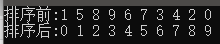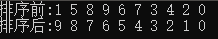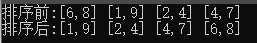# C++ sort 排序及自定义排序## 默认排序

#include<bits/stdc++.h>using namespace std;int main(){    int arr[] = { 1, 5, 8, 9, 6, 7, 3, 4, 2, 0 };    int n = sizeof(arr) / sizeof(arr);    cout << "排序前:" ;    for (int i = 0; i < n; i++)    {        cout << arr[i] << " ";    }    cout <<endl;    cout << "排序后:";    sort(arr, arr + n);    for (int i = 0; i < n; i++)    {        cout << arr[i] << " ";    }    cout <<endl;    return 0;}## 降序排序

greater<int>() 说明 ， greater 意为更大

<?> 可以为 默认类型，array，int， string 等类型

#include<bits/stdc++.h>using namespace std;int main(){    int arr[] = { 1, 5, 8, 9, 6, 7, 3, 4, 2, 0 };    int n = sizeof(arr) / sizeof(arr);    cout << "排序前:" ;    for (int i = 0; i < n; i++)    {        cout << arr[i] << " ";    }    cout <<endl;    cout << "排序后:";    sort(arr, arr + n, greater<int>());    for (int i = 0; i < n; i++)    {        cout << arr[i] << " ";    }    cout <<endl;    return 0;}## 自定义排序

#include<bits/stdc++.h>using namespace std;struct Interval{    int startInt;    int endInt;};bool cmp(Interval a, Interval b){    return a.startInt < b.startInt;}int main(){    Interval arr[]    = { { 6, 8 }, { 1, 9 }, { 2, 4 }, { 4, 7 } };    int n = sizeof(arr) / sizeof(arr);    cout << "排序前:" ;    for (int i = 0; i < n; i++)        cout << "[" << arr[i].startInt << "," << arr[i].endInt             << "] ";    cout <<endl;    cout << "排序后:";    sort(arr, arr + n, cmp);    for (int i = 0; i < n; i++)        cout << "[" << arr[i].startInt << "," << arr[i].endInt             << "] ";    cout <<endl;    return 0;}## 评论